# Switching regression axes

Gold Member
Yes, this is correct.

@FactChecker can confirm, but I don’t think that he is disagreeing with me. He is just showing you why the two choices are not equivalent.
I do disagree. How accurately a variable can be measured is not the significant issue. The head/tail result of a coin toss can be measured with great accuracy but that does not make that result the independent variable. The decision of whether to model Y=aX+b+##\epsilon## versus X=a'Y+b'+##\epsilon##' is a matter of how you will use the data, what SSE you want to minimize, and whether you want the standard statistical theory and results to apply to your use. How the data will be used should determine which linear regression to do.
It's essential to be minimizing the correct errors. The regression of X as a linear function of Y is guaranteed to minimize ##\sum (x_i-\hat {x_i})^2##.
The two approaches are easy to compare. Just do both regressions and see which one has the smaller SSE for that sample using ##y_i## to estimate ##x_i##.

Last edited:
•Dale

Dale
Mentor
2020 Award
How accurately a variable can be measured is not the significant issue. ...
The two approaches are easy to compare. Just do both regressions and see which one has the smaller SSE for that sample using ##y_i## to estimate ##x_i##.
Indeed, so let's do a Monte Carlo simulation and see. I used ##x## going from 0 to 1 in steps of 0.01, with a true regression of ##y=2 x + 5##. I added zero-mean Gaussian white noise to both x and y, and ran two linear regressions one for the model ##y= a x + b + \epsilon## and the other for the model ##x = a' y + b' + \epsilon## which I then inverted to get an estimate for ##y=a x + b##.

First, I set ##\sigma_x=0.01## and ##\sigma_y=0.5##. Then the first fit gave ##y=1.90 x + 5.03## and after the inversion the second fit gave ##y=3.54x+4.20##. In this case the first fit gave regression coefficients much closer to the true values.

Second, I set ##\sigma_x=0.5## and ##\sigma_y=0.01##. Then the first fit gave ##y=0.54 x + 5.78## and after the inversion the second fit gave ##y=1.61 x + 5.33##. In this case the second fit gave regression coefficients closer to the true values.

In both cases, the best regression was obtained when the "noisier" variable was the one modeled. So when ##\sigma_x > \sigma_y## the better model was ##x = a' y + b' + \epsilon##, even though the resulting fit had to be inverted to use as desired.

Last edited:
WWGD
Gold Member
There are ultimately formulas that allow us to obtain E( Y|X) from E(X|Y). Maybe we can use them to estimate E( y|X=xo) from E(x| Y=yo)?

Stephen Tashi
. In this case the second fit gave regression coefficients closer to the true values.
Is the problem to minimize the square error of the estimated regression coefficients? - or is it to minimize the square error of predictions of the given y data from the given x data?

•FactChecker
Gold Member
Indeed, so let's do a Monte Carlo simulation and see. I used ##x## going from 0 to 1 in steps of 0.01, with a true regression of ##y=2 x + 5##. I added zero-mean Gaussian white noise to both x and y, and ran two linear regressions one for the model ##y= a x + b + \epsilon## and the other for the model ##x = a' y + b' + \epsilon## which I then inverted to get an estimate for ##y=a x + b##.

First, I set ##\sigma_x=0.01## and ##\sigma_y=0.5##. Then the first fit gave ##y=1.90 x + 5.03## and after the inversion the second fit gave ##y=3.54x+4.20##. In this case the first fit gave regression coefficients much closer to the true values.

Second, I set ##\sigma_x=0.5## and ##\sigma_y=0.01##. Then the first fit gave ##y=0.54 x + 5.78## and after the inversion the second fit gave ##y=1.61 x + 5.33##. In this case the second fit gave regression coefficients closer to the true values.

In both cases, the best regression was obtained when the "noisier" variable was the one modeled. So when ##\sigma_x > \sigma_y## the better model was ##x = a' y + b' + \epsilon##, even though the resulting fit had to be inverted to use as desired.
For a given simulated data set, ##(x_i, y_i)##, see which regression line gives the better SSE, ##\sum (x_i-\hat{x_i})^2)##. Unless the regression algorithm is flawed, it must be the line obtained from the X=a'Y+b' regression line because that is the minimization that the regression algorithm for X=a'Y+b' does. The Y=aX+b regression line is minimizing the wrong thing. It is minimizing ##\sum (y_i-\hat{y_i})^2)##.

PS. If you simulate a certain model and change the criteria for which approach is "best", then the test may be rigged so that the "better" result is of the simulated form.

Gold Member
There are ultimately formulas that allow us to obtain E( Y|X) from E(X|Y). Maybe we can use them to estimate E( y|X=xo) from E(x| Y=yo)?
Just to be clear. For any given ##x_0## you may have little or no sample data at or near that value. So you must specify a model and a form of the model equation that allows you to use a large number of your sample data to get an estimate at ##x_0##. That is what you get from the linear regression line.

Dale
Mentor
2020 Award
The Y=aX+b regression line is minimizing the wrong thing.
Why is that the wrong thing? If most of your errors are in Y then you get a better result minimizing that.

Gold Member
Why is that the wrong thing? If most of your errors are in Y then you get a better result minimizing that.
It's wrong because the goal is to estimate X. If you use a model that is worse (sometimes very much worse) on the sample data, then you can expect it to be worse for the intended use.

Stephen Tashi
Why is that the wrong thing? If most of your errors are in Y then you get a better result minimizing that.

It's easy to agree with that on an intuitive level, but I think it's challenging to formulate that thought rigorously. How is the "result" quantified?

For example, if we assert ##y = Ax + B## and we mis-estimate ##A## by 0.5 and mis-estimate ##B## by 0.2 then is the result (0.5)(0.5) + (0.2)(0.2) ? - and is it the same result as mis-estimating ##A## by 0.2 and ##B## by 0.5 ?

Dale
Mentor
2020 Award
It's wrong because the goal is to estimate X. If you use a model that is worse (sometimes very much worse) on the sample data, then you can expect it to be worse for the intended use.
But it isn’t worse. See the Monte Carlo results above.

Dale
Mentor
2020 Award
I think it's challenging to formulate that thought rigorously
I definitely agree with that. And this isn’t something that the usual diagnostics check.

The bigger point is that one of the assumptions of the OLS regression is that the independent variables have 0 error. In practice that is never true but “close enough” is fine. Sometimes you can get “close enough” by flipping your variables, and sometimes you need completely different techniques. But simply ignoring a large violation of this assumption can cause problems, as shown above.

Gold Member
But it isn’t worse. See the Monte Carlo results above.
I read that post but did not see anything about how well the alternatives did at estimating x values. By the definition of the regression algorithm, the linear regression for the model X = aY+b will minimize ##\sum (x_i-\hat {x_i})^2##. I consider anything else to be worse. The other regression might appear better in some respects because your simulation model was of a matching form, but that is not a valid test.

Dale
Mentor
2020 Award
I read that post but did not see anything about how well the alternatives did at estimating x values
Often the goal is to estimate the model coefficients. Particularly when those coefficients have some known meaning.

Gold Member
Often the goal is to estimate the model coefficients. Particularly when those coefficients have some known meaning.
It is probably an advantage to estimate the parameters of the correct model. You used a Y=aX+b+##\epsilon## model to generate data in a simulation and then the Y=aX+b linear regression performed better at parameter estimation. I would have to think about that. But for the OP data, is there any logical reason to pick that model, not even knowing what the data is from?
In general, if you are trying to get the line, ##\hat{X}=a'Y+b'##, that best estimates X based on Y from a set of ##(x_i,y_i)## data, then it is better to minimize the correct thing, which is ##\sum (x_i-\hat{x_i})^2##, not ##\sum (y_i-\hat{y_i})^2##.

Last edited:
•Dale
Hard to see in practice how there would be confusion around the dependent and independent variable

It is not necessarily the more volatile one that is LH- for example, if you have an individual stock and the S&P 500, you regress the stock return against the index return, even though its possible (but not likely) that the standard deviation of the stock is less than the index.

Dale
Mentor
2020 Award
In general, if you are trying to get the line, ##\hat{X}=a'Y+b'##, that best estimates X based on Y from a set of ##(x_i,y_i)## data, then it is better to minimize the correct thing, which is ##\sum (x_i-\hat{x_i})^2##, not ##\sum (y_i-\hat{y_i})^2##.
Again, you can just test that sort of claim by running a Monte Carlo simulation. So, similar to what I did before, consider the true values of ##y## going from 0 to 1 in steps of 0.01 and the true values of ##x=2y+5##. I then added 0 mean Gaussian white noise to ##x## and ##y## with ##\sigma_x=0.01## and ##\sigma_y=0.5##. Next I did two fits, a "forward" fit of ##x=a y + b + \epsilon## and an "inverse" fit of ##y = a' x + b'+ \epsilon## where the desired fit parameters were then determined by ##a=1/a'## and ##b=-b'/a'##. I repeated this process 10000 times.

So, if we look at the sum of square residuals on the data. We see that indeed as you have stated the forward fit has a substantially smaller sum of squared residuals to the data.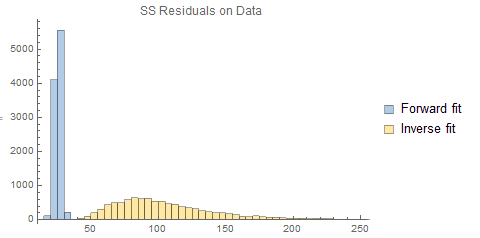However, if we look at the sum of squares residuals to the true regression line we see a very different outcome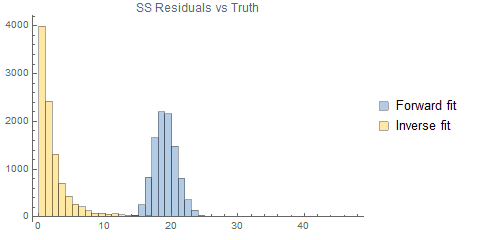So the forward fit is closer to the data, but the inverse fit is closer to the true relationship in a least-squares sense. In other words, it is fitting to the noise rather than to the actual relationship.

More importantly, if we look at the fit parameters we see that for both the slope and the intercept parameters, the forward fit is rather strongly biased whereas the inverse fit parameters appear unbiased.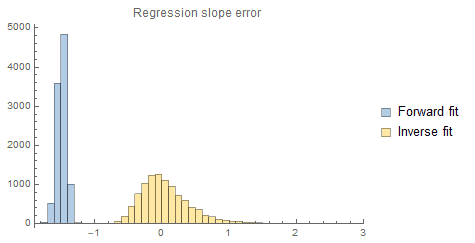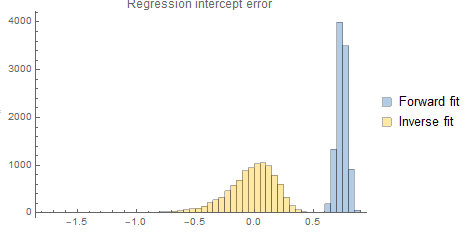Finally, we can compare the fit lines with the true regression. Notice how reliably wrong the forward fit is.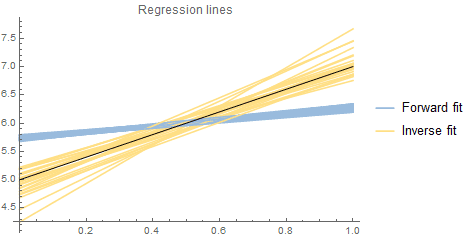So the forward fit is the "best estimate" only in one very narrow sense. However, that does not mean that it is generally a better choice.

The issue is that the narrow sense in which it is better relies on an assumption which is strongly violated because ##\sigma_y## is so large. With the violation of this assumption the usual fit is no longer an unbiased minimum varisnce estimator. It is therefore better to switch to the inverse model which does not violate the assumption. Even though the resulting fits are suboptimal in the narrow sense, they are better under a much broader set of criteria and importantly the parameter estimates are unbiased.

Another alternative is to use an "errors in variables" model that does not make the assumption that the "independent" variable has no errors. But as we see, when one variable approximately satisfies the assumption then you can use that one and a standard least-squares fit and then invert the model.

Last edited:
Gold Member
This gives me food for thought. If you do not know ahead of time that the data came from a simulation of Y = aX+b, how can a person distinguish which is the best regression to use either for parameter estimation or for estimations of X from Y?

•Dale
Dale
Mentor
2020 Award
how can a person distinguish which is the best regression to use either for parameter estimation or for estimations of X from Y?
If one parameter (X in the recent example) has a small standard deviation and the other does not, then the accurate one should serve as the predictor. Again, fundamentally this is about checking the validity of the model assumptions.

Gold Member
If one parameter (X in the recent example) has a small standard deviation and the other does not, then the accurate one should serve as the predictor. Again, fundamentally this is about checking the validity of the model assumptions.
It surprises me that there is a significant difference. Modeling ##Y = aX+b+\epsilon## is the same as modeling ##X=(1/a)Y-b/a-\epsilon/a##, both linear regression problems. And it seems like the difference in the standard deviation is just a matter of the range of values and the units of measurement for the two variables, neither of which should really matter. So I don't see where one can be better due to that.

But I do see the advantage of minimizing the correct SSE, ##\sum (x_i-\hat{x_i})^2##.

Last edited:
Dale
Mentor
2020 Award
But I do see the advantage of minimizing the correct SSE, ##\sum (x_i-\hat{x_i})^2##.
How can you rationalize that claim after the above demonstration? The evidence shows that it is clearly disadvantageous.

The fact that the resulting estimates are biased is a death-knell. If a technique is unbiased but not minimum variance, then you simply need more data to get a good estimate and improve the variance. But if a technique is biased then no amount of additional data will fix it.

It is not "correct" to use a technique whose assumptions are violated, even if doing so minimizes some variance.

And it seems like the difference in the standard deviation is just a matter of the range of values and the units of measurement for the two variables, neither of which should really matter. So I don't see where one can be better due to that.
And yet, a Monte Carlo simulation easily shows that it is better. Assumptions are important in statistics.

Gold Member
How can you rationalize that claim after the above demonstration? The evidence shows that it is clearly disadvantageous.

The fact that the resulting estimates are biased is a death-knell. If a technique is unbiased but not minimum variance, then you simply need more data to get a good estimate and improve the variance. But if a technique is biased then no amount of additional data will fix it.

It is not "correct" to use a technique whose assumptions are violated, even if doing so minimizes some variance.

And yet, a Monte Carlo simulation easily shows that it is better. Assumptions are important in statistics.
Minimizing the correct errors is minimizing the correct errors. If your simulation analysis shows otherwise, then it is seriously flawed.
Your test did not analyze the effect of the range and units of measurement of the two variables. The decision of which regression to use should be agnostic of scale and units of measure. But I can mathematically see that both directly influence, perhaps dominate, your recommended decision.

Gold Member
Suppose the true model is ##X=100Y+\epsilon##. Then for each ##(x_i, y_i, \epsilon_i)## we have ##x_i=100*y_i + \epsilon_i## and ##y_i = x_i/100 + \epsilon_i/100##. So clearly, the SD of the sample ##y_i##s is orders of magnitude smaller than the ##x_i##s. Your recommendation is that the regression should be with Y as the dependent variable and X independent. That will be minimizing the wrong SSE. I don't think that can be justified.

Last edited:
Dale
Mentor
2020 Award
Minimizing the correct errors is minimizing the correct errors.
How can you justify calling a biased minimization “correct”? What is “correct” about bias?

That will be minimizing the wrong SSE. I don't think that can be defended.
I am not defending that. As I said in post 11 I agree that it is challenging to formulate this idea rigorously. I don't know of a standard test for making this decision. So I do not advocate ignorant or blind decision. I am merely pointing out that the decision requires considering the validity of the "zero error" assumption. The result of that consideration may be that the "inverse" approach is actually the better choice.

The decision of which regression to use should be agnostic of scale and units of measure.
That is not generally true in statistics.

Last edited:
Gold Member
How can you justify calling a biased minimization “correct”? What is “correct” about bias?

I am not defending that. As I said in post 11 I agree that it is challenging to formulate this idea rigorously. I don't know of a standard test for making this decision. So I do not advocate ignorant or blind decision. I am merely pointing out that the decision requires considering the validity of the "zero error" assumption. The result of that consideration may be that the "inverse" approach is actually the better choice.

That is not generally true in statistics.
Here is one consequence of your recommendation.
Suppose we have an experiment of temperatures versus associated positions. Your recommendation would likely change depending on whether the temperatures were measured in Fahrenheit or Celsius and whether the positions were measured in inches, feet, or yards.
I do not like that. If you think that is right, I guess we will just have to agree to disagree.

Last edited:
Dale
Mentor
2020 Award
Here is one consequence of your recommendation.
Suppose we have an experiment of temperatures versus associated positions. Your recommendation would likely change depending on whether the temperatures were measured in Fahrenheit or Celsius and whether the positions were measured in inches, feet, or yards.
I do not like that. If you think that is right, I guess we will just have to agree to disagree.
This is a strawman. As I have stated 3 times now it is challenging to formulate this issue rigorously, and I know of no formal test for it. So I am not advocating a blind rule like your strawman.

I guess we will just have to agree to disagree.
Ok, but the evidence is pretty clear: strong enough violations of the assumption will introduce bias. That much is not a matter of opinion.

The matter of opinion is only whether or not it is acceptable to choose a biased estimator when an unbiased estimator is available.

Last edited: